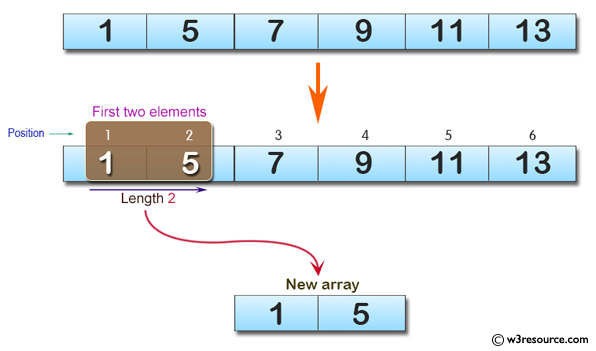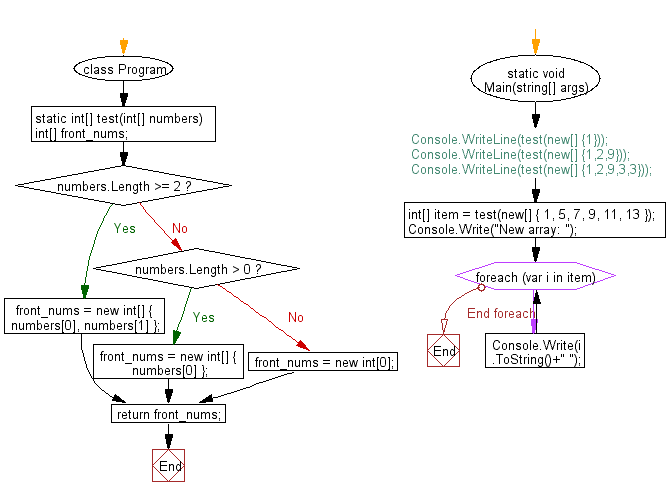﻿ C# - New array taking the first two elements from a array# C# Sharp Basic Algorithm Exercises: Create a new array taking the first two elements from a given array

## C# Sharp Basic Algorithm: Exercise-108 with Solution

Write a C# Sharp program to create a new array taking the first two elements from a given array. If the length of the given array is less than 2 then return the give array.

Pictorial Presentation:Sample Solution:-

C# Sharp Code:

``````using System;
namespace exercises
{
class Program
{
static void Main(string[] args)
{
//  Console.WriteLine(test(new[] {1}));
//  Console.WriteLine(test(new[] {1,2,9}));
//  Console.WriteLine(test(new[] {1,2,9,3,3}));

int[] item = test(new[] { 1, 5, 7, 9, 11, 13 });
Console.Write("New array: ");
foreach(var i in item)
{
Console.Write(i.ToString()+" ");
}

}
static int[] test(int[] numbers)
{
int[] front_nums;

if (numbers.Length >= 2)
{
front_nums = new int[] { numbers, numbers };
}
else if (numbers.Length > 0)
{
front_nums = new int[] { numbers };
}
else
{
front_nums = new int;
}

return front_nums;
}
}
}
```
```

Sample Output:

`New array: 1 5`

Flowchart:C# Sharp Code Editor:

Improve this sample solution and post your code through Disqus

What is the difficulty level of this exercise?

Test your Programming skills with w3resource's quiz.

﻿